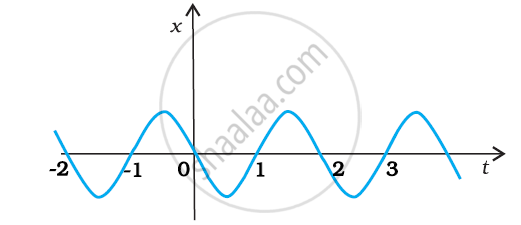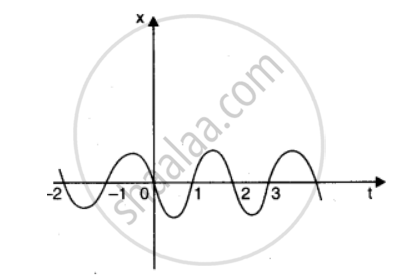CBSE (Science) Class 11CBSE
Share

# Figure Gives The X-t Plot of a Particle Executing One-dimensional Simple Harmonic Motion. Give the Signs of Position, Velocity and Acceleration Variables of the Particle At T = 0.3 S, 1.2 S, – 1.2 S. - CBSE (Science) Class 11 - Physics

ConceptKinematic Equations for Uniformly Accelerated Motion

#### Question

Figure gives the x-t plot of a particle executing one-dimensional simple harmonic motion. Give the signs of position, velocity and acceleration variables of the particle at = 0.3 s, 1.2 s, – 1.2 s.#### Solution 1

Negative, Negative, Positive (at t = 0.3 s)

Positive, Positive, Negative (at t = 1.2 s)

Negative, Positive, Positive (at t = –1.2 s)

For simple harmonic motion (SHM) of a particle, acceleration (a) is given by the relation:

a = – ω2x ω → angular frequency … (i)

t = 0.3 s

In this time interval, x is negative. Thus, the slope of the x-t plot will also be negative. Therefore, both position and velocity are negative. However, using equation (i), acceleration of the particle will be positive.

t = 1.2 s

In this time interval, x is positive. Thus, the slope of the x-t plot will also be positive. Therefore, both position and velocity are positive. However, using equation (i), acceleration of the particle comes to be negative.

t = – 1.2 s

In this time interval, x is negative. Thus, the slope of the x-t plot will also be negative. Since both xand t are negative, the velocity comes to be positive. From equation (i), it can be inferred that the acceleration of the particle will be positive.

#### Solution 2

In x-t graph of Fig. showing simple harmonic motion of a particle, the signs of position, velocity and acceleration are as given below.In S.H.M., acceleration, a – x or a = – kx.

(i) At t = 0.3 s, x < 0 i.e., x is in -ve direction. Moreover, as x is becoming more negative with time, it shows that v is also – ve (i.e., v < 0). However, a = -kx will be +ve (a > 0).

(ii) At t = 1.2 s, x > 0, v > 0 and a < 0.

(iii) At t = -1.2 s, x < 0, but here on increasing the time t, value of x becomes less negative.

It means that v is +ve (i.e., v > 0). Again a = – kx will be positive (i.e., a > 0).

Is there an error in this question or solution?

#### APPEARS IN

NCERT Solution for Physics Textbook for Class 11 (2018 to Current)
Chapter 3: Motion in a Straight Line
Q: 20 | Page no. 58
Solution Figure Gives The X-t Plot of a Particle Executing One-dimensional Simple Harmonic Motion. Give the Signs of Position, Velocity and Acceleration Variables of the Particle At T = 0.3 S, 1.2 S, – 1.2 S. Concept: Kinematic Equations for Uniformly Accelerated Motion.
S